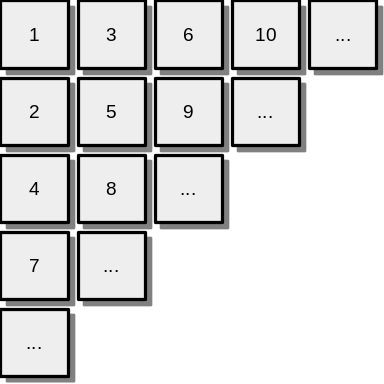# Infinitely Large GridhasibhossainIntra AUST Programming Co...
Limits 1s, 512 MB

One day Joti, Risty and Mithy — three friends were playing with an infinitely large grid together. The columns of the grid are numbered from left to right starting from 1 and the rows are counted from top to bottom in the similar way. There were positive integers written in each tile of the grid. All the integers in the grid are unique and appear in a beautiful manner as shown in the following image:The game is played as— Joti picks a positive integer N and asks her friends to find the position of N in the grid. Then Risty needs to find out the row R and Mithy needs to find out the column C of the grid where N is written. For example— if Joti picks 9, the answer will be “2 3“. If she picks 1, the answer will be “1 1“. Help Risty and Mithy finding their answers to T different test cases.

## Input

First line of the input contains a single integer T $(1\le T \le 10^5)$ denoting the number of test cases.

Each of the next T lines contains a single positive integer N $(1\le N \le 10^9)$ denoting the number picked by Joti in that test case.

## Output

For each of the queries, output two integers R and C in a single line denoting the answer to that query.

## Sample

InputOutput
4
3
6
8
12

1 2
1 3
3 2
4 2


### Submit